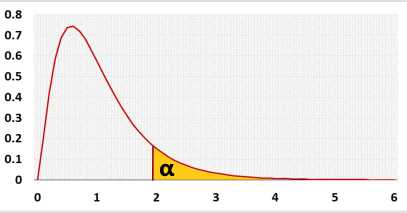# Sample size calculator

## Linear regression, ANOVA (F distribution)

 distribution: RegressionPower analysis for the entire model

## Information

Calculates the sample size basted on the number of predictors and draw a power analysis chart. Use this test for one of the following tests:
Simple Linear Regression
Multiple Linear Regression
One Way ANOVA

 f2 = R2 ,   R2 = f2 1 - R2 1 + f2

Example:
Linear regression with 4 predictors, α=0.05, power=0.8.
A sample of 85 will identify model with R2=0.13. (or f=0.3873 or f2=0.15)
i.e. the power of a model with a smaller R2 will be lower than 0.8 .

### Distribution## R Code

The following R code should produce the same results